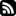Posts Downloads Tags About# Indie Recursion

Recursion is at the heart of programming. Recursion allows a function to call itself, making it possible to define small functions that work on data of unbounded sizes. Loops are also a form of recursion, just in disguise.

A simple example of a recursive function is the factorial. We can define it recursively for example in Scheme and JavaScript as:

```function fakt(x) {if (x == 0) return 1; return x * fakt(x - 1); }
fakt(10) // -> 3628800
```
```(define (fakt x) (if (= x 0) 1 (* x (fakt (- x 1)))))
(fakt 10) ;; -> 3628800
```

Ignore the fact that this isn't an efficient way to compute it. We just need a recursive function as an example.

Now, instead of using a builtin way of defining a function, we can naturally just define the variable to have a function as a value.

```var fakt = function(x) {if (x == 0) return 1; return x * fakt(x - 1); }
fakt(10) // -> 3628800
```
```(define fakt (lambda (x) (if (= x 0) 1 (* x (fakt (- x 1))))))
(fakt 10) ;; -> 3628800
```

We know what the function is, so we could now call it directly without assigning it to a variable. That would seem like a valid thing to do. The trouble is, that in case the function is a recursive one, you can't do that. Can you see why?

Recursion usually works in programming languages via a hack. First we reserve space from memory from a fixed location where the recursive function(s) will end up being stored. Then we compile the function, with the knowledge where it shall be stored in the future. Whenever a recursive call needs to be compiled, we know where the value can be loaded from. It's just not there yet.

For a concept that is at the very heart of programming, this seems like a rather hacky solution.

Could we solve this some other way? Of course we can. You may have already heard about the Y-combinator, which computes fixed points of functions. Though a useful theoretical construction, it's not especially useful in practice. We need control over arity, ability to handle mutual recursion, and we want code that we can optimize and run efficiently.

Let's take the previously mentioned function call attempts, and convert them using a technique developed for owl for this purpose:

```(function(x) {if (x == 0) return 1; return x * fakt(x - 1); })(10);
// -> error
```
```((lambda (x) (if (= x 0) 1 (* x (fakt (- x 1))))) 10)
;; -> error
```

First step: Find all unbound calls. In this case fakt is the only unbound one. Assume all of these to be recursive mutually dependent functions.

Second step: Introduce a new internal function(s), which have the same arguments and body as the original one(s), but which also receive all the previously computed dependencies as arguments.

Third step: Add all the new arguments passed to the internal function to call sites of the recursive dependencies.

Last step (optional): Define wrapper functions after the newly added internal ones, which have the same arguments as the original functions. Body of these functions is just the call the to corresponding internal one with its dependencies.

```(function(x) {
let sub = function(x, fakt) {
if (x == 0) return 1;
return x * fakt(x - 1, fakt);};
let fakt = function(x) { return sub(x, sub); };
return fakt(x);})(10);
// 3628800
```
```((lambda (x)
(let* ((sub (lambda (x fakt)
(if (= x 0) 1
(* x (fakt (- x 1) fakt)))))
(fakt (lambda (x) (sub x sub))))
(fakt x)))
10)
;; -> 3628800
```

This transformation is easy to implement as a separate compiler step. Notice that the resulting code involves no assignments, so it should be easy for subsequent optimization steps to handle.

Posted: 17.10.2020 by aoh